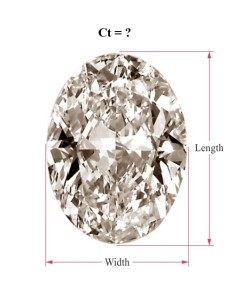Gemstones

# Guide : How to Determine the Carat Weight of Oval Diamonds by Measurements

Soualy Guide : How to Determine the Carat Weight of Oval Diamonds by Measurements

If you have an oval diamond and want to determine its carat weight but don’t have a way to weigh the stone, you can make an estimate. To do so, you can use a formula that calculates an approximate carat weight from the measurements of the stone, or you can use a conversion table that shows what carat value certain measurements correspond to.

## Estimating the Weight of an Oval Diamond Using a FormulaThe weight of an oval-cut diamond can be estimated using the following formula:

Carat Weight = Diameter x Diameter x Depth x 0.006

If you are wondering how the diameter of an oval stone should be calculated for the purpose of this formula, here’s the way it works: Just add the width and the length of the stone, measured at its widest and longest part respectively, and divide the result by two.

For example, an oval stone with measurements of 6 mm and 4 mm will have a diameter of (6 + 4)/2 = 5 mm.

The depth of a diamond is the distance from its top to its bottom (the bottom is defined as the point, or the single facet, farthest from the top).

So, if an oval diamond has a depth of 3 mm and a diameter of 5 mm, its estimated weight will be calculated as follows:

5 mm x 5 mm x 3 mm x 0.006 = 0.45 carats

This calculation is not very precise, but it is good enough to give you an idea of the approximate weight of a diamond.

In the above example, it is recommended that you interpret the result in terms of a range, i.e., the diamond’s true weight should be assumed to be 0.45 ct +/- 10%, or somewhere between 0.40 and 0.50 carats.

## Millimeter-to-Carat Conversion Table for Oval Diamonds

In the table below, you can see how some of the most common oval diamond measurements convert to carat weights.

On the left, you have combinations of length and width, in millimeters.

On the right, you have the corresponding estimated carat values for the respective measurements:

5.0 x 3.0 mm – 0.25 ct

6.0 x 4.0 mm – 0.50 ct

7.5 x 5.0 mm – 0.75 ct

8.0 x 5.5 mm – 1.00 ct

8.5 x 5.5 mm – 1.25 ct

9.0 x 6.0 mm – 1.50 ct

10.5 x 7.0 mm – 2.00 ct

11.5 x 7.5 mm – 3.00 ct

Keep in mind that this conversion table assumes a fixed depth value for each length-width combination, so the actual carat weight for each measurement may vary depending on the actual depth of the stone.

This is why you should use the table only to get an approximate idea of where your stone stands in terms of weight and use the oval diamond weight formula to get a more precise estimate.

## Where to Buy Diamond Jewelry?

We recommend James Allen (read review) because you can see a 360-degree video for any diamond before buying it.

Thank you 🙂 Soualy.com Best 925 Sterling Silver Jewelry : rings, pendants, earrings, bracelets, ..